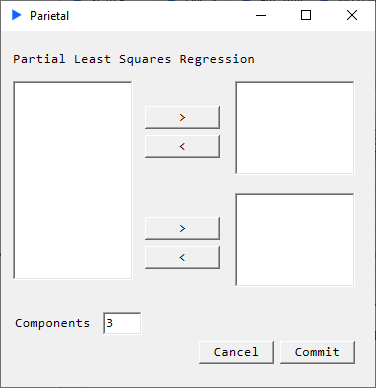# Partial Least Squares Regression

PLSR is used to find relationships between two matrices and is primarily used for predictive purposes. More generally, it is a technique that reduces a set of variables to a smaller (user specified) set of orthogonal components and performs least squares regression on these components. The model produces a 'percentage variance explained' metric for each of the components in both the matrices which can give the analyst information regarding how many components to specify.##### Description#

The model in PLSR can be described as: $$X = X_{scores} * X_{loading}^\mathrm{T} + E$$ $$Y = Y_{scores} * Y_{loading}^\mathrm{T} + F$$

##### Returns#

The relationships between the returned variables are described below.

• X Loadings: $X_{loading} = X_{centered}^{'} * X_{scores}$
• Y Loadings: $Y_{loading} = Y_{centered}^{'} * X_{scores}$
• X Scores
• Y Scores
• Beta: $Y = [Ones,X]*Beta + F$
• % Var: Row 1 containes the percentage variance explained in X by each component. Same for row 2 for Y.
• Weights: $X_{scores} = X_{centered} * W$
• T2: Hotelling t-squared statistic as a generalization of Student’s t-statistic for $X_{scores}$
• X Residuals: $X_{centered} - X_{scores} * X_{loading}^{'}$
• Y Residuals: $Y_{centered} - X_{scores} * Y_{loading}^{'}$